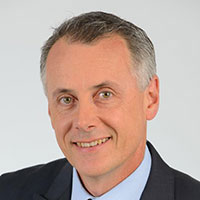#### this is titleTom Gellibrand
Deputy Program Director for Metro Product and Integration
Transport for New South Wales, Sydney

oen two three four five six oen two three four five six oen two three four five six oen two three four five six oen two three four five six oen two three four five six oen two three four five six oen two three four five six oen two three four five six oen two three four five six oen two three four five six oen two three four five six oen two three four five six oen two three four five six oen two three four five six oen two three four five six oen two three four five six oen two three four five six oen two three four five six oen two three four five six oen two three four five six oen two three four five six ur five six oen two three four five six oen two three four five six oen two three four five six oen two three four five six oen two three four five six oen two three four five six oen two three four five six oen two three four five six oen two three four five six oen two three four five six oen two three four five six oen two three four five six oen two three four five six oen two three four five six oen two three four five six oen two three four five six oen two three four five six oen two three four five six oen two three four five six oen two three four five six oen two three four five six